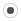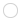This forum will soon be archived and you won't be able to interact with it anymore. Please use KDE Discuss instead.

## MappedSparseMatrix with SPQRpjsa
Registered MemberPosts
15
Karma
0

### MappedSparseMatrix with SPQR

Thu Sep 19, 2013 1:11 am
Hi,
When I try to solve using SPQR constructed with a MappedSparseMatrix the compilater fails:
eigen/Eigen/src/SPQRSupport/SuiteSparseQRSupport.h:142:12: error: no matching function for call to ‘Eigen::Map<Eigen::Matrix<double, -1, 1>, 0, Eigen::Stride<0, 0> >::Map()
//Compute Q^T * b
Dest y;
y = matrixQ().transpose() * b;

I noticed that SparseQR has a different version of this operation, which is ok with the map
// Compute Q^T * b;
typename Dest::PlainObject y, b;
y = this->matrixQ().transpose() * B;
b = y;

This is with the development branch.

thanks!pjsa
Registered MemberPosts
15
Karma
0

### Re: MappedSparseMatrix with SPQR

Thu Sep 19, 2013 8:31 pm
Also, I was wondering if I call compute more than once on the same solver object (e.g. SPQR or any other) passing a different matrix in each case then can I rely on eigen to not leak memory? Or must I always delete the solver object and construct a new one for each matrix to be factored? In the case of SPQR it is leaking memory.

I also have noticed a few issues with other sparse solvers:
1. Compiler error (gcc 4.7.3) with the constructor CholmodDecomposition(const MatrixType& matrix). The fix is to replace compute(matrix) with Base::compute(matrix) .
2. UmfPackLU doesn't work with the MappedSparseMatrix<double,ColMajor,int>, I need to copy it into a SparseMatrix first or the code crashes in _solve.Dee33
Registered MemberPosts
54
Karma
0
OS### Re: MappedSparseMatrix with SPQR

Tue Sep 24, 2013 1:44 pm
pjsa wrote:When I try to solve using SPQR constructed with a MappedSparseMatrix the compilater fails:
eigen/Eigen/src/SPQRSupport/SuiteSparseQRSupport.h:142:12: error: no matching function for call to ‘Eigen::Map<Eigen::Matrix<double, -1, 1>, 0, Eigen::Stride<0, 0> >::Map()

Hi,
I cannot reproduce this error. Could you give a small example which produces this error.
Note that your error is related with Eigen::Map and not Eigen::MappedSparseMatrix.Dee33
Registered MemberPosts
54
Karma
0
OS### Re: MappedSparseMatrix with SPQR

Tue Sep 24, 2013 1:58 pm
pjsa wrote:Also, I was wondering if I call compute more than once on the same solver object (e.g. SPQR or any other) passing a different matrix in each case then can I rely on eigen to not leak memory? Or must I always delete the solver object and construct a new one for each matrix to be factored? In the case of SPQR it is leaking memory.

It is now fixed in the devel branch
https://bitbucket.org/eigen/eigen/commits/01def857d4f2/
Changeset: 01def857d4f2
User: dnuentsa
Date: 2013-09-24 15:56:56
Summary: Fix leaked memory for successive calls to SPQR
Affected #: 1 fileDee33
Registered MemberPosts
54
Karma
0
OS### Re: MappedSparseMatrix with SPQR

Tue Sep 24, 2013 2:06 pm
pjsa wrote:2. UmfPackLU doesn't work with the MappedSparseMatrix<double,ColMajor,int>, I need to copy it into a SparseMatrix first or the code crashes in _solve.

Please, check how you build your MappedSparseMatrix. There is no problem on my side to use UmfPackLU with it.

Here is my small test code
Code: Select all
`UmfPackLU<SparseMatrix<double, ColMajor> > solver;SparseMatrix<double, ColMajor> A; // Fill A as usual  MappedSparseMatrix<double, ColMajor, int> B(A.rows(), A.cols(), A.nonZeros(), A.outerIndexPtr(), A.innerIndexPtr(), A.valuePtr());solver.compute(B); `pjsa
Registered MemberPosts
15
Karma
0

### Re: MappedSparseMatrix with SPQR

Tue Sep 24, 2013 6:31 pm

First, regarding the umfpack issue, here is a small test problem that generates the error. In this case I am not building the mapped sparse matrix the same way as in my large-scale application but I think it is the same issue because when i run with valgrind the errors are the same in both cases. I am using g++ 4.7.3 and umfpack 5.4.0 on ubuntu 13.4 with the dev branch of eigen. If this code doesn't crash try running it with valgrind.

The issue is that I am using SparseMatrix<double, ColMajor> as the template argument when instantiating the UmfPackLU object. When I use MappedSparseMatrix<double, ColMajor, int> instead, it works fine. Some of the other solvers that I have tried (e.g. cholmod) work the opposite way to umfpack, i.e. the template argument must be SparseMatrix.

Code: Select all
`// compiled with: g++ -I/usr/include/suitesparse -I./eigen  -lumfpack sparse.cpp#include <Eigen/Core>#include <Eigen/Sparse>#include <Eigen/UmfPackSupport>#include <iostream>int main(){   using namespace Eigen;   SparseMatrix<double, ColMajor> A(10,10);   for(int i=0; i<10; ++i) A.insert(i,i) = 1;   A.makeCompressed();   std::cerr << "A =\n" << A << std::endl;   MappedSparseMatrix<double, ColMajor, int> B(A.rows(), A.cols(), A.nonZeros(), A.outerIndexPtr(), A.innerIndexPtr(), A.valuePtr());   UmfPackLU<SparseMatrix<double, ColMajor> > solver;   solver.compute(B);   Matrix<double,10,1> x = Matrix<double,10,1>::Random();   std::cerr << "x        = " << x.transpose() << std::endl;   std::cerr << "solution = " << solver.solve(x).transpose() << std::endl;}`

Last edited by pjsa on Tue Sep 24, 2013 8:20 pm, edited 1 time in total.pjsa
Registered MemberPosts
15
Karma
0

### Re: MappedSparseMatrix with SPQR

Tue Sep 24, 2013 7:41 pm
Here is the same example with cholmod, only passing the mapped matrix to the constructor instead of calling compute. This fails to compile with g++ 4.7.3. My understanding is that some compilers will accept this but it is not conforming to the standard.
the compiler error is:
In file included from ./eigen/Eigen/CholmodSupport:39:0,
from sparse2.cpp:4:
./eigen/Eigen/src/CholmodSupport/CholmodSupport.h: In instantiation of ‘Eigen::CholmodDecomposition<_MatrixType, _UpLo>::CholmodDecomposition(const MatrixType&) [with _MatrixType = Eigen::SparseMatrix<double, 0>; int _UpLo = 2; Eigen::CholmodDecomposition<_MatrixType, _UpLo>::MatrixType = Eigen::SparseMatrix<double, 0>]’:
sparse2.cpp:21:68: required from here
./eigen/Eigen/src/CholmodSupport/CholmodSupport.h:534:7: error: ‘compute’ was not declared in this scope, and no declarations were found by argument-dependent lookup at the point of instantiation [-fpermissive]
./eigen/Eigen/src/CholmodSupport/CholmodSupport.h:534:7: note: declarations in dependent base ‘Eigen::CholmodBase<Eigen::SparseMatrix<double, 0>, 2, Eigen::CholmodDecomposition<Eigen::SparseMatrix<double, 0>, 2> >’ are not found by unqualified lookup

Also, I noticed that valgrind gives uninitialized value warnings on this problem.

Code: Select all
`// compile with: g++ -I/usr/include/suitesparse -I./eigen -lcholmod sparse.cpp#include <Eigen/Core>#include <Eigen/Sparse>#include <Eigen/CholmodSupport>#include <iostream>int main(){   using namespace Eigen;   SparseMatrix<double, ColMajor> A(10,10);   for(int i=0; i<10; ++i) A.insert(i,i) = 1;   A.makeCompressed();   std::cerr << "A =\n" << A << std::endl;   MappedSparseMatrix<double, ColMajor, int> B(A.rows(), A.cols(), A.nonZeros(), A.outerIndexPtr(), A.innerIndexPtr(), A.valuePtr());   CholmodDecomposition<SparseMatrix<double>,Eigen::Upper> solver(B);   Matrix<double,10,1> x = Matrix<double,10,1>::Random();   std::cerr << "x        = " << x.transpose() << std::endl;   std::cerr << "solution = " << solver.solve(x).transpose() << std::endl;}`

Last edited by pjsa on Tue Sep 24, 2013 8:21 pm, edited 1 time in total.pjsa
Registered MemberPosts
15
Karma
0

### Re: MappedSparseMatrix with SPQR

Tue Sep 24, 2013 8:19 pm
Finally, getting back to the original post about SPQR. As you pointed the issue is not with the use of MappedSparseMatrix. It is because I am evaluating the solution into a Map. Here is an example that fails to compile. You can refer to the SparseQR _solve function for the correction.

Code: Select all
`// compile with: g++ -I/usr/include/suitesparse -I./eigen sparse.cpp -lspqr -lcholmod -lblas -llapack#include <Eigen/Core>#include <Eigen/Sparse>#include <Eigen/SPQRSupport>#include <iostream>int main(){   using namespace Eigen;   SparseMatrix<double, ColMajor> A(10,10);   for(int i=0; i<10; ++i) A.insert(i,i) = 1;   A.makeCompressed();   std::cerr << "A =\n" << A << std::endl;   MappedSparseMatrix<double, ColMajor, int> B(A.rows(), A.cols(), A.nonZeros(), A.outerIndexPtr(), A.innerIndexPtr(), A.valuePtr());   SPQR<SparseMatrix<double,ColMajor> > solver;   solver.compute(B);   Matrix<double,10,1> x = Matrix<double,10,1>::Random();   double data;   Map<Matrix<double,10,1> > solution(&data);   solution = solver.solve(x);   std::cerr << "x        = " << x.transpose() << std::endl;   std::cerr << "solution = " << solution.transpose() << std::endl;}`ggael
ModeratorPosts
3447
Karma
19
OS### Re: MappedSparseMatrix with SPQR

Wed Oct 02, 2013 9:53 pm
I don't have time to look into it but I guess the issue come from missing overloads for the conversions from/to Eigen's type to/from suitesparse types for MappedSparseMatrix. Don't be afraid of looking at the source code of these modules to see if you can fix them yoursefl!! Also makes sure you did not compiled with -DNEBUG as some functions might have prerequisites on storage order of the inputs.